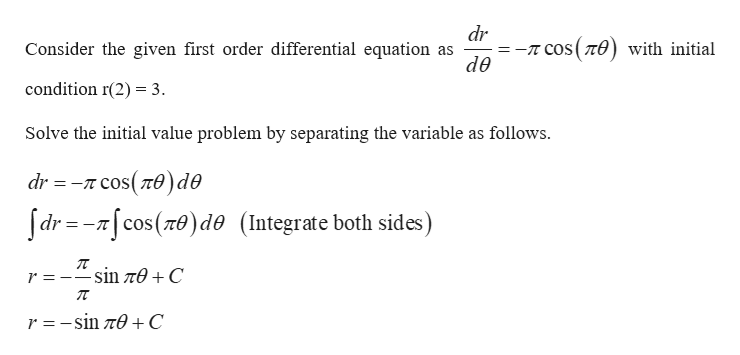# solve the following initial value problem.dr/dθ= -πcos πθ, r(2)=3(Type an exact answer, using π as needed.)

Question
3 views

solve the following initial value problem.

dr/dθ= -πcos πθ, r(2)=3

(Type an exact answer, using π as needed.)

check_circle

Step 1help_outlineImage Transcriptionclosedr -T COS(T) with initial Consider the given first order differential equation as de condition r(2) = 3 Solve the initial value problem by separating the variable as follows πcos( πθ) dθ dr =-cos(n0)de (Integrate both sides) r -sin Te C r =-sin r0 +C fullscreen

### Want to see the full answer?

See Solution

#### Want to see this answer and more?

Solutions are written by subject experts who are available 24/7. Questions are typically answered within 1 hour.*

See Solution
*Response times may vary by subject and question.
Tagged in

### Differential Equations